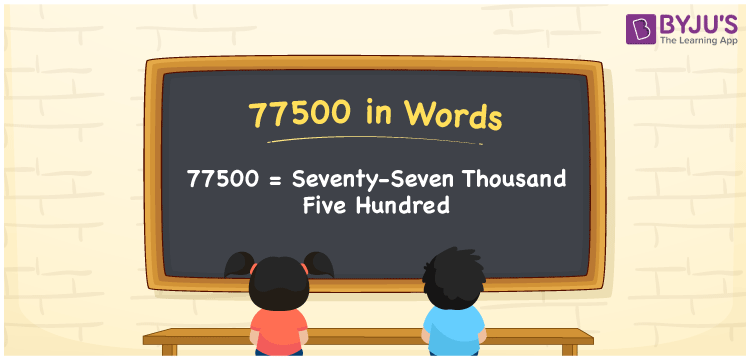# 77500 in words

77500 in words is written as Seventy Seven Thousand Five Hundred. In 77500, the first 7 has a place value of ten thousand, second 7 is in the place value of thousand and 5 is in the place value of hundred. The article on Place Value gives more information. The number 77500 is used in expressions that relate to money, distance, social media views, and many more. For example, “Your Youtube channel has Seventy Seven Thousand Five Hundred subscribers.”

 77500 in words Seventy Seven Thousand Five Hundred Seventy Seven Thousand Five Hundred in Numbers 77500

## 77500 in English Words## How to Write 77500 in Words?

We can convert 77500 to words using a place value chart. The number 77500 has 5 digits, so let’s make a chart that shows the place value up to 5 digits.

 Ten thousand Thousands Hundreds Tens Ones 7 7 5 0 0

Thus, we can write the expanded form as:

7 × Ten thousand + 7 × Thousand + 5 × Hundred + 0 × Ten + 0 × One

= 7 × 10000 + 7 × 1000 + 5 × 100 + 0 × 10 + 0 × 1

= 77500.

= Seventy Seven Thousand Five Hundred.

77500 is the natural number that is succeeded by 77499 and preceded by 77501.

77500 in words – Seventy Seven Thousand Five Hundred.

Is 77500 an odd number? – No.

Is 77500 an even number? – Yes.

Is 77500 a perfect square number? – No.

Is 77500 a perfect cube number? – No.

Is 77500 a prime number? – No.

Is 77500 a composite number? – Yes.

## Solved Example

1. Write the number 77500 in expanded form

Solution: 7 x 10000 + 7 x 1000 + 5 x 100 + 0 x 10 + 0 x 1

We can write 77500 = 70000 + 7000 + 500 + 00 + 0

= 7 x 10000 + 7 x 1000 + 5 x 100 + 0 x 10 + 0 x 1.

## Frequently Asked Questions on 77500 in words

Q1

### How to write the number 77500 in words?

77500 in words is written as Seventy Seven Thousand Five Hundred.
Q2

### Is 77500 a prime number?

No. 77500 is not a prime number.
Q3

### Is 77500 divisible by 10?

Yes. 77500 is divisible by 10.